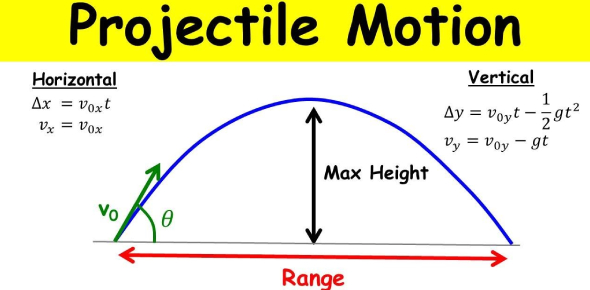# The Projectile Motion Quiz! Trivia

10 Questions | Total Attempts: 8031SettingsProjectile motion is a type of motion caused by an object being dropped to the earth's surface. The force that is the cause of this motion is gravity. The object first goes upwards then is forced to come downwards. In this quiz, you will get to test out just how much you know about this type of motion and how to measure its velocity.

• 1.
Projectile motion has two components, horizontal (x) and vertical (y) as can be seen below. The horizontal motion has:
• A.

Constant acceleration

• B.

Changing acceleration

• C.

Constant velocity

• D.

Changing velocity

• E.

Both velocity and acceleration

• 2.
A _____________ is any object moving only under the influence of gravity.
• 3.
Two objects are released from the same height. Object A falls straight down from the cliff and object B follows a curved parabolic path. It is true that:
• A.

A hits the ground before B

• B.

B hits the ground before A

• C.

A hits the ground first only if it is heavier than B

• D.

A and B hit the ground at the same time.

• 4.
A ball is projected vertically upwards with an initial velocity of 50 m.s-1. Using the formula 'v = u + at' the calculated velocity after 2s would be?
• A.

-30.4 m.s-1

• B.

30.4 m.s-1

• C.

-69.6 m.s-1

• D.

69.6 m.s-1

• 5.
If an object is not moving there is no force acting upon it.
• A.

True

• B.

False

• 6.
Acceleration can be defined as the rate at which an object changes its position.
• A.

True

• B.

False

• 7.
The mass of a bowling ball on the moon is the same as the mass of a bowling ball on Earth.
• A.

True

• B.

False

• 8.
The path of a projectile is known as its _________________
Related TopicsBack to top